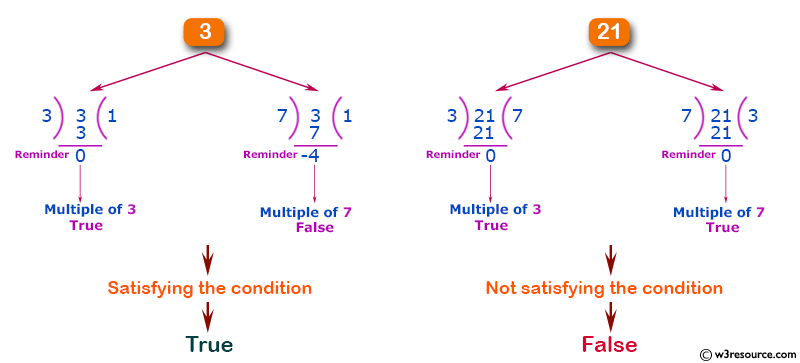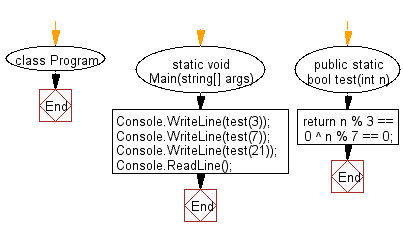﻿ C# - Non-negative numbers multiples of 3 or 7, but not both# C# Sharp Basic Algorithm Exercises: Check whether a given non-negative given number is a multiple of 3 or 7, but not both

## C# Sharp Basic Algorithm: Exercise-43 with Solution

Write a C# Sharp program to check whether a given non-negative given number is a multiple of 3 or 7, but not both.

Pictorial Presentation:Sample Solution:-

C# Sharp Code:

``````using System;
using System.Linq;
namespace exercises
{
class Program
{
static void Main(string[] args)
{
Console.WriteLine(test(3));
Console.WriteLine(test(7));
Console.WriteLine(test(21));
}
public static bool test(int n)
{
return n % 3 == 0 ^ n % 7 == 0;
}
}
}
```
```

Sample Output:

```True
True
False```

Flowchart:C# Sharp Code Editor:

Improve this sample solution and post your code through Disqus

What is the difficulty level of this exercise?

Test your Programming skills with w3resource's quiz.

﻿